Click to Chat

1800-1023-196

+91-120-4616500

CART 0

• 0

MY CART (5)

Use Coupon: CART20 and get 20% off on all online Study Material

ITEM
DETAILS
MRP
DISCOUNT
FINAL PRICE
Total Price: Rs.

There are no items in this cart.
Continue Shopping• Complete Physics Course - Class 11
• OFFERED PRICE: Rs. 2,968
• View Details

```Refraction at a Plane Surface

(i) The incident ray, the refracted ray and the normal to the refracting surface at the point of incidence all lie in the same plane.
(ii) The ratio of the sines of the angle of incidence (i) and of the angle of refraction (r) is a constant quantityμfor two given media, which is called the refractive index of the second medium with respect to the first.

(sin i / sin r) = constant = μ

When light propagates through a series of layers of different medium as shown in the figure, then the Snell’s law may be written as
μ1sin 1 = μ2sin 2 = μ3sin 3 = μ4sin 4 = constant
In general, μ sinθ = constant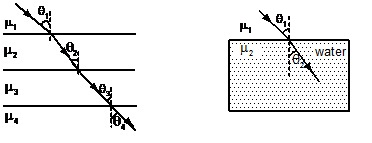Fig a (series of transparent layers of different refractive indices), Fig b (A light ray passing from air to water bends toward the normal)

When light passes from rarer to denser medium it bends toward the normal as shown in the fig.
According to Snell’s law
μ1sin θ1 = μ2sin θ2
When a light ray passes from denser to rarer medium it bends away from the normal as shown in the fig. b above

For a given point object, the image formed by refraction at plane surface is illustrated by the following diagrams.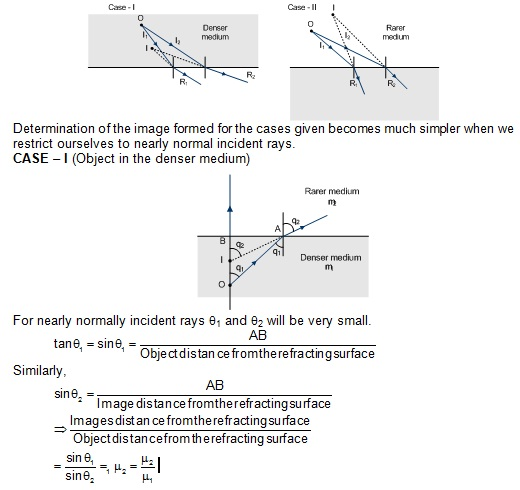The same result is obtained for the other case also. The image distance from the refracting surface is also known as Apparent depth or height.
Apparent Shift
Apparent shift = Object distance from refracting surface – image distance from refracting surface.

Δy (apparent shift) t = 1 - μ-1 where t is the object distance and μ = μ1/ μ2
• If there are a number of slabs with different refractive indices placed between the observer and the object.
Total apparent shift = ΣΔyi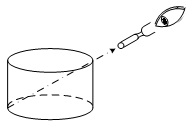Illustration 3: A person looking through a telescope T just sees the point A on the rim at the bottom of a cylindrical vessel when the vessel is empty. When the vessel is completely filled with a liquid (? = 1.5), he observes a mark at the centre, B, of the vessel. What is the height of the vessel if the diameter of its cross-section is 10cm?

Solution: It is mentioned in the problem that on filling the vessel with the liquid, point B is observed for the same setting; this means that the images of point B, is observed at A, because of refraction of the ray at C.

For refraction at C :sin r / sin i = μ1= 1.5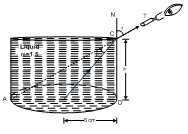sin r =AD / AC = 10/ √( 102+ h2), where h is height of vessel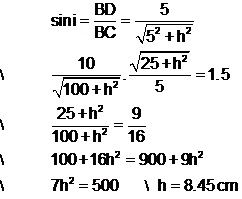To read more, Buy study materials of Ray Optics and Optical Instruments comprising study notes, revision notes, video lectures, previous year solved questions etc. Also browse for more study materials on Physics here.

April Fools Day is coming. Prank your friends opening a never ending fake update screen on their computer. Sit back and watch their reaction.
```### Course Features

• 101 Video Lectures
• Revision Notes
• Previous Year Papers
• Mind Map
• Study Planner
• NCERT Solutions
• Discussion Forum
• Test paper with Video Solution